# Newton’s laws of motionPage 3

#### WATCH ALL SLIDES

A. also 40 m/s.

45 m/s.

50 m/s.

none of the above.

Newton’s Second Law of Motion

Slide 19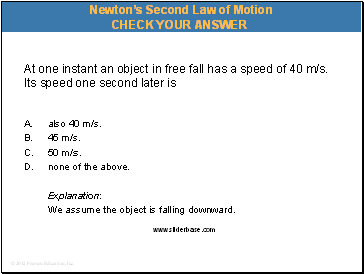At one instant an object in free fall has a speed of 40 m/s. Its speed one second later is

A. also 40 m/s.

45 m/s.

50 m/s.

none of the above.

Explanation:

We assume the object is falling downward.

Newton’s Second Law of Motion

Slide 20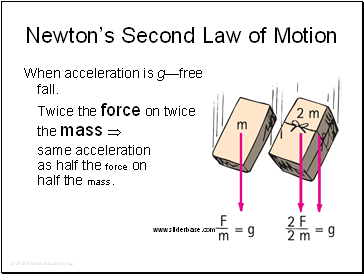Newton’s Second Law of Motion

When acceleration is g—free fall.

Twice the force on twice the mass 

same acceleration as half the force on half the mass.

Slide 21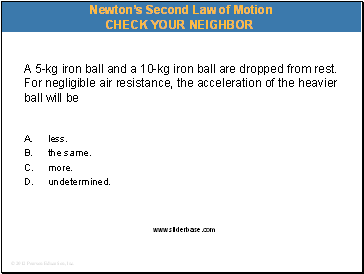A 5-kg iron ball and a 10-kg iron ball are dropped from rest. For negligible air resistance, the acceleration of the heavier ball will be

A. less.

the same.

more.

undetermined.

Newton’s Second Law of Motion

Slide 22A 5-kg iron ball and a 10-kg iron ball are dropped from rest. For negligible air resistance, the acceleration of the heavier ball will be

A. less.

the same.

more.

undetermined.

Newton’s Second Law of Motion

Slide 23A 5-kg iron ball and a 10-kg iron ball are dropped from rest. When the free-falling 5-kg iron ball reaches a speed of 10 m/s, the speed of the free-falling 10-kg iron ball is

A. less than 10 m/s.

10 m/s.

more than 10 m/s.

undetermined.

Newton’s Second Law of Motion

Slide 24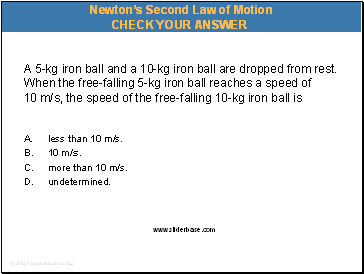A 5-kg iron ball and a 10-kg iron ball are dropped from rest. When the free-falling 5-kg iron ball reaches a speed of 10 m/s, the speed of the free-falling 10-kg iron ball is

A. less than 10 m/s.

10 m/s.

more than 10 m/s.

undetermined.

Newton’s Second Law of Motion

Slide 25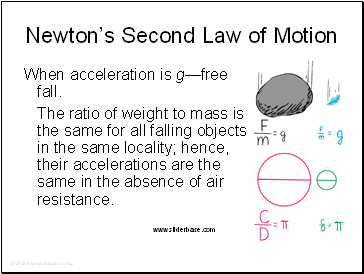Newton’s Second Law of Motion

When acceleration is g—free fall.

The ratio of weight to mass is the same for all falling objects in the same locality; hence, their accelerations are the same in the absence of air resistance.

Slide 26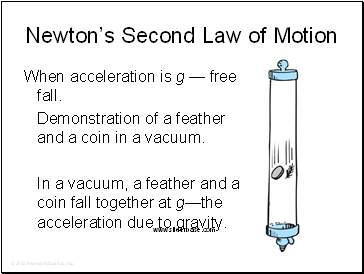Newton’s Second Law of Motion

When acceleration is g — free fall.

Demonstration of a feather and a coin in a vacuum.

In a vacuum, a feather and a coin fall together at g—the acceleration due to gravity.

Go to page:
1  2  3  4  5  6  7  8  9